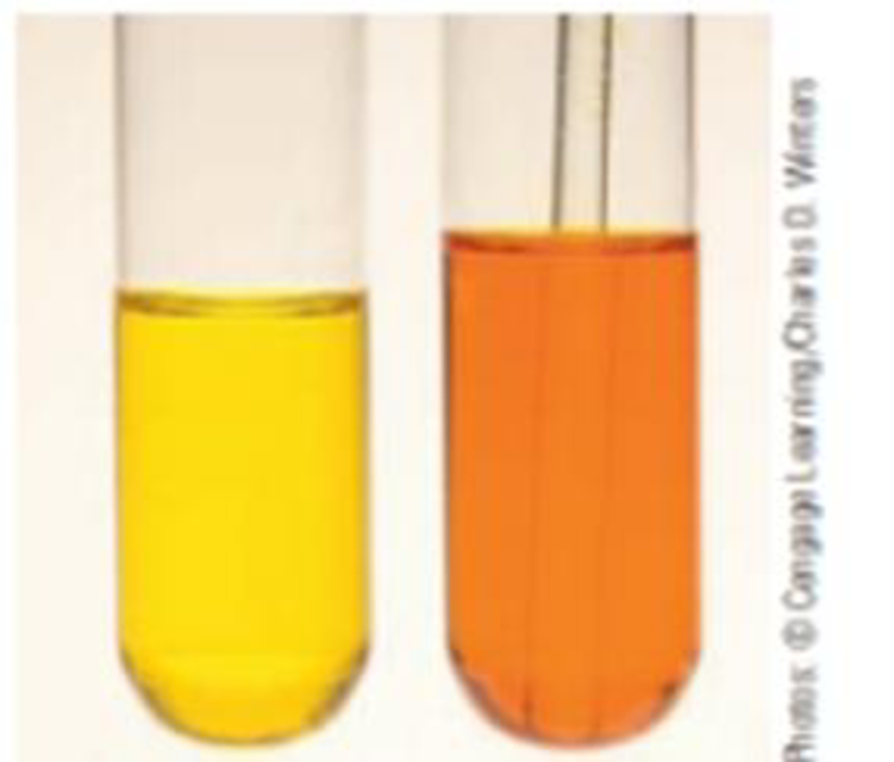Chapter 15, Problem 66IL

Chapter
Section
Textbook Problem

The photograph below shows what occurs when a solution of potassium chromate is treated with a few drops of concentrated hydrochloric acid. Some of the bright yellow chromate ion is converted to the orange dichromate ion.2 CrO42−(aq) + 2 H3O+(aq) ⇄ CrO72−(aq) + 3 H2O(ℓ)(a) Explain this experimental observation in terms of Le Chatelier’s principle.(b) What would you observe if you treated the orange solution with sodium hydroxide? Explain your observation.

(a)

Interpretation Introduction

Interpretation:

The conversion of bright yellow chromate ion to orange dichromate ion when a solution of potassium dichromate is treated with few drops of hydrochloric acid has to be explained in terms of Le Chatelier’s principle.

Concept Introduction:

Le Chatelier’s principle: If equilibrium is disturbed by changing conditions, the system will moves the equilibrium to reverse the change.

Factor’s that effect chemical equilibria:

• Concentration – Equilibrium will be affected by changing the concentration of reactant or product. If we increase the concentration of reactant system will try to reverse the change by favouring forward reaction and thus increase the concentration of products. Likewise adding products increase yield of reactants.
• Temperature – When the temperature increases equilibrium will shift in the endothermic direction, in the direction that absorbs heat. When the temperature decreases equilibrium will shift in the exothermic direction, in the direction that releases heat.
• Pressure – If the reaction consists of only liquid and solid reactants and products, pressure has no effect in the equilibrium.

In gas reactions if the number of moles has no change then there will be no effect by pressure on equilibrium.

If pressure increases then equilibrium will shift to the direction having less number of molecules and if pressure decreases system will shift to the direction having more number of molecules.

Explanation

Given reaction:

2CrO42-(aq)+2H3O+(aq)Cr2O72-(aq)+3H2O(l)

The equilibrium between chromate ion and dichromate can be explained by the given reaction

(b)

Interpretation Introduction

Interpretation:

The observation when the yellow solution is treated with sodium hydroxide has to be explained.

Concept Introduction:

Le Chatelier’s principle: If equilibrium is disturbed by changing conditions, the system will moves the equilibrium to reverse the change.

Factor’s that effect chemical equilibria:

• Concentration – Equilibrium will be affected by changing the concentration of reactant or product. If we increase the concentration of reactant system will try to reverse the change by favouring forward reaction and thus increase the concentration of products. Likewise adding products increase yield of reactants.
• Temperature – When the temperature increases equilibrium will shift in the endothermic direction, in the direction that absorbs heat. When the temperature decreases equilibrium will shift in the exothermic direction, in the direction that releases heat.
• Pressure – If the reaction consists of only liquid and solid reactants and products, pressure has no effect in the equilibrium.

In gas reactions if the number of moles has no change then there will be no effect by pressure on equilibrium.

If pressure increases then equilibrium will shift to the direction having less number of molecules and if pressure decreases system will shift to the direction having more number of molecules.

Still sussing out bartleby?

Check out a sample textbook solution.

See a sample solution

The Solution to Your Study Problems

Bartleby provides explanations to thousands of textbook problems written by our experts, many with advanced degrees!

Get Started